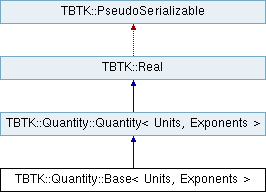TBTK Need a break? Support the development by playing Polarity PuzzlesTBTK::Quantity::Base< Units, Exponents > Class Template Reference

#include <Base.h>

Inheritance diagram for TBTK::Quantity::Base< Units, Exponents >:## Public Types

using IsBaseQuantity = std::true_typePublic Types inherited from TBTK::Quantity::Quantity< Units, Exponents >
using Unit = Units

using Exponent = Exponents

## Friends

void initializeBaseQuantities ()

## Related Functions

(Note that these are not member functions.)

typedef Base< AngleUnit, AngleExponent > Angle

typedef Base< ChargeUnit, ChargeExponent > Charge

typedef Base< CountUnit, CountExponent > Count

typedef Base< EnergyUnit, EnergyExponent > Energy

typedef Base< LengthUnit, LengthExponent > Length

typedef Base< TemperatureUnit, TemperatureExponent > Temperature

typedef Base< TimeUnit, TimeExponent > TimePublic Member Functions inherited from TBTK::Quantity::Quantity< Units, Exponents >
Quantity ()

Quantity (double value)Public Member Functions inherited from TBTK::Real
Real ()

constexpr Real (double value)

Real (const std::string &serialization, Serializable::Mode mode)

constexpr operator double () const

Realoperator= (double rhs)

Realoperator+= (const Real &rhs)

Realoperator-= (const Real &rhs)

Realoperator*= (const Real &rhs)

Realoperator/= (const Real &rhs)

std::string serialize (Serializable::Mode mode) constStatic Public Member Functions inherited from TBTK::Quantity::Quantity< Units, Exponents >
static std::string getUnitString (Unit unit)

static Unit getUnit (const std::string &unit)

static double getConversionFactor (Unit unit)Static Protected Attributes inherited from TBTK::Quantity::Quantity< Units, Exponents >
static class TBTK::Quantity::Quantity::ConversionTable conversionTable

## Detailed Description

### template<typename Units, typename Exponents> class TBTK::Quantity::Base< Units, Exponents >

The Base Quantity is a Quantity with the compile time directive IsBaseQuantity set to std::true_type to differentiate it from Derived Quantities. The Base Quantity is instantiated by the seven Base Quantities Angle, Charge, Count, Energy, Length, Temperature, and Time. For more information, see Quantity and the individual typedefs below.

## ◆ Angle

template<typename Units , typename Exponents >
 typedef Base Angle
related

The Quantity::Angle is a Quantity::Base with the following predefined base units

• Quantity::Angle::Unit::degree

## ◆ Charge

template<typename Units , typename Exponents >
 typedef Base Charge
related

The Quantity::Charge is a Quantity::Base with the following predefined base units

• Quantity::Charge::Unit::kC
• Quantity::Charge::Unit::C
• Quantity::Charge::Unit::mC
• Quantity::Charge::Unit::uC
• Quantity::Charge::Unit::nC
• Quantity::Charge::Unit::pC
• Quantity::Charge::Unit::fC
• Quantity::Charge::Unit::aC
• Quantity::Charge::Unit::Te
• Quantity::Charge::Unit::Ge
• Quantity::Charge::Unit::Me
• Quantity::Charge::Unit::ke
• Quantity::Charge::Unit::e

## ◆ Count

template<typename Units , typename Exponents >
 typedef Base Count
related

The Quantity::Count is a Quantity::Base with the following predefined base units

• Quantity::Count::Unit::pcs
• Quantity::Count::Unit::mol

## ◆ Energy

template<typename Units , typename Exponents >
 typedef Base Energy
related

The Quantity::Energy is a Quantity::Base with the following predefined base units

• Quantity::Energy::Unit::GeV
• Quantity::Energy::Unit::MeV
• Quantity::Energy::Unit::keV
• Quantity::Energy::Unit::eV
• Quantity::Energy::Unit::meV
• Quantity::Energy::Unit::ueV
• Quantity::Energy::Unit::J

## ◆ initializeBaseQuantities

template<typename Units , typename Exponents >
 void initializeBaseQuantities ( )
friend

Initialize the Base Quantities.

## ◆ Length

template<typename Units , typename Exponents >
 typedef Base Length
related

The Quantity::Length is a Quantity::Base with the following predefined base units

• Quantity::Length::Unit::m
• Quantity::Length::Unit::mm
• Quantity::Length::Unit::um
• Quantity::Length::Unit::nm
• Quantity::Length::Unit::pm
• Quantity::Length::Unit::fm
• Quantity::Length::Unit::am
• Quantity::Length::Unit::Ao

## ◆ Temperature

template<typename Units , typename Exponents >
 typedef Base Temperature
related

The Quantity::Temperature is a Quantity::Base with the following predefined base units

• Quantity::Temperature::Unit::kK
• Quantity::Temperature::Unit::K
• Quantity::Temperature::Unit::mK
• Quantity::Temperature::Unit::uK
• Quantity::Temperature::Unit::nK

## ◆ Time

template<typename Units , typename Exponents >
 typedef Base Time
related

The Quantity::Time is a Quantity::Base with the following predefined base units

• Quantity::Time::Unit::s
• Quantity::Time::Unit::ms
• Quantity::Time::Unit::us
• Quantity::Time::Unit::ns
• Quantity::Time::Unit::ps
• Quantity::Time::Unit::fs
• Quantity::Time::Unit::as

The documentation for this class was generated from the following file:
• /home/kristofer.bjornson/SecondQuantizationCom/TBTK/Lib/include/TBTK/Quantity/Base.h